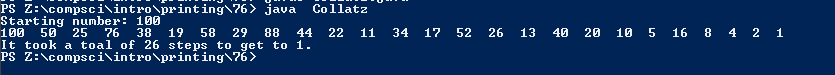PROGRAMMING AND CODE

# Assignemnt #76

## Code

```/// Name: Justin Li
/// Period: 7
/// Program Name: Collatz
/// File Name: Collatz.java
/// Date Completed: 2/11/16

import java.util.Scanner;

public class Collatz {

public static void main(String[] args) throws Exception {

Scanner keyboard = new Scanner(System.in);

int n, n1;
n1 = 1;

System.out.print("Starting number: ");
n = keyboard.nextInt();

System.out.print(n);

if (n != 1 && n > 0) {

while (n != 1) {
if (n % 2 == 0)
n = n/2;
else if (n % 2 == 1)
n = ((n*3)+1);
System.out.print("  " + n);
n1++;
}
System.out.println("\nIt took a toal of " + n1 + " steps to get to 1.");
}
else
System.out.println("What did you do");

}
}
```

### Picture of the output